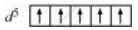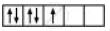Share

# [Fe(H2O)6]3+ is strongly paramagnetic whereas [Fe(CN)6]3− is weakly paramagnetic. Explain. - CBSE (Science) Class 12 - Chemistry

ConceptBonding in Coordination Compounds Magnetic Properties of Coordination Compounds

#### Question

[Fe(H2O)6]3+ is strongly paramagnetic whereas [Fe(CN)6]3− is weakly paramagnetic. Explain.

#### Solution

In both [Fe(H_2O)_6]^(3+) and [Fe(CN)_6]^(3-) Fe exists in the +3 oxidation state i.e., in d5 configuration.Since CN is a strong field ligand, it causes the pairing of unpaired electrons. Therefore, there is only one unpaired electron left in the d-orbital.Therefore,

mu = sqrt(n(n+2))

=sqrt(1(1+2))

=sqrt3

=1.732 BM

On the other hand, H2O is a weak field ligand. Therefore, it cannot cause the pairing of electrons. This means that the number of unpaired electrons is 5.

Therefore,

mu = sqrt(n(n+2))

=sqrt(5(5+2))

=sqrt35

=6 BM

Thus it is evident that [Fe(H_2O)_6]^(3+) is strongly paramagnetic, while [Fe(CN)_6]^(3-)is weakly paramagnetic

Is there an error in this question or solution?

#### APPEARS IN

NCERT Solution for Chemistry Textbook for Class 12 (2018 to Current)
Chapter 9: Coordinate Compounds
Q: 7 | Page no. 254
Solution [Fe(H2O)6]3+ is strongly paramagnetic whereas [Fe(CN)6]3− is weakly paramagnetic. Explain. Concept: Bonding in Coordination Compounds - Magnetic Properties of Coordination Compounds.
S